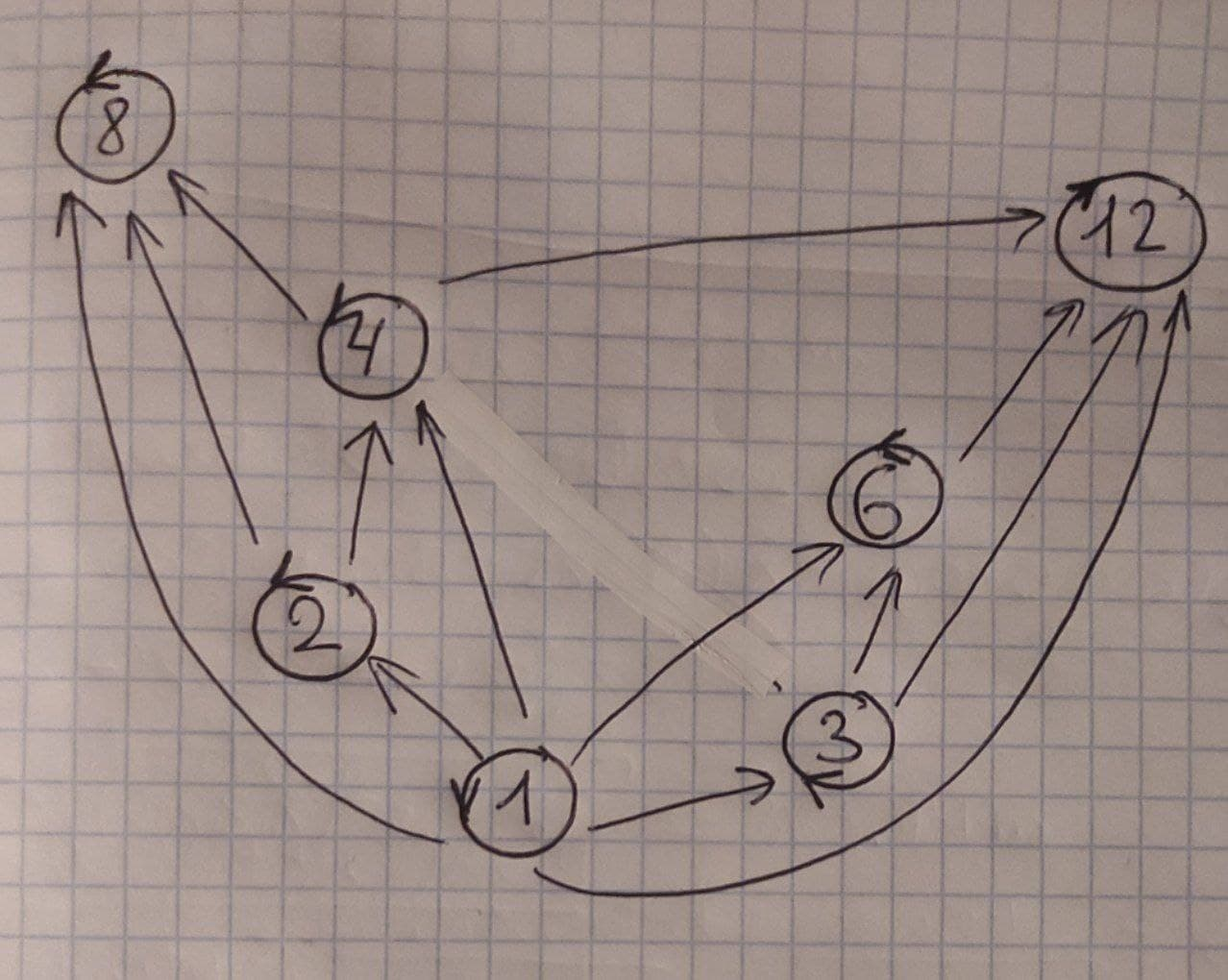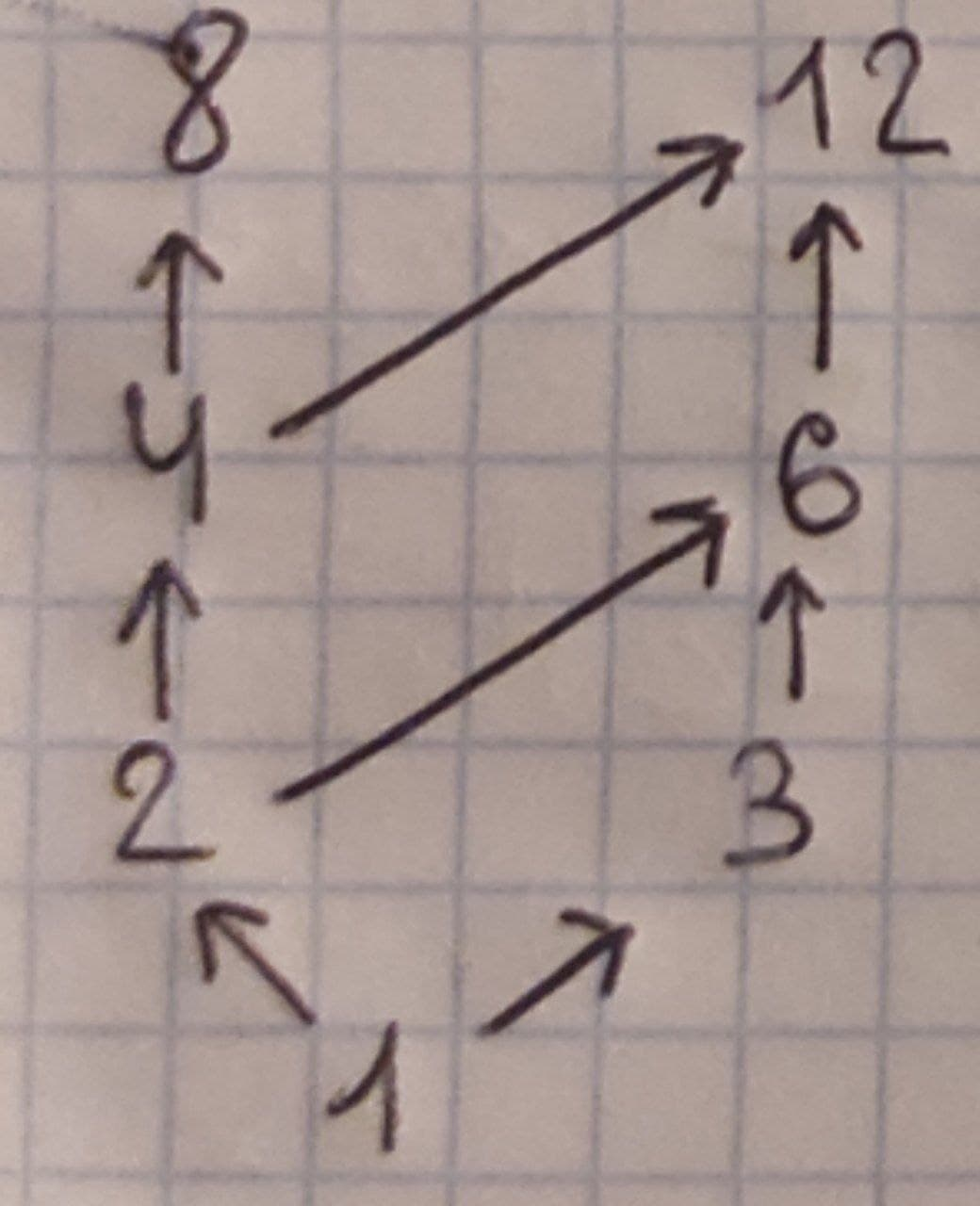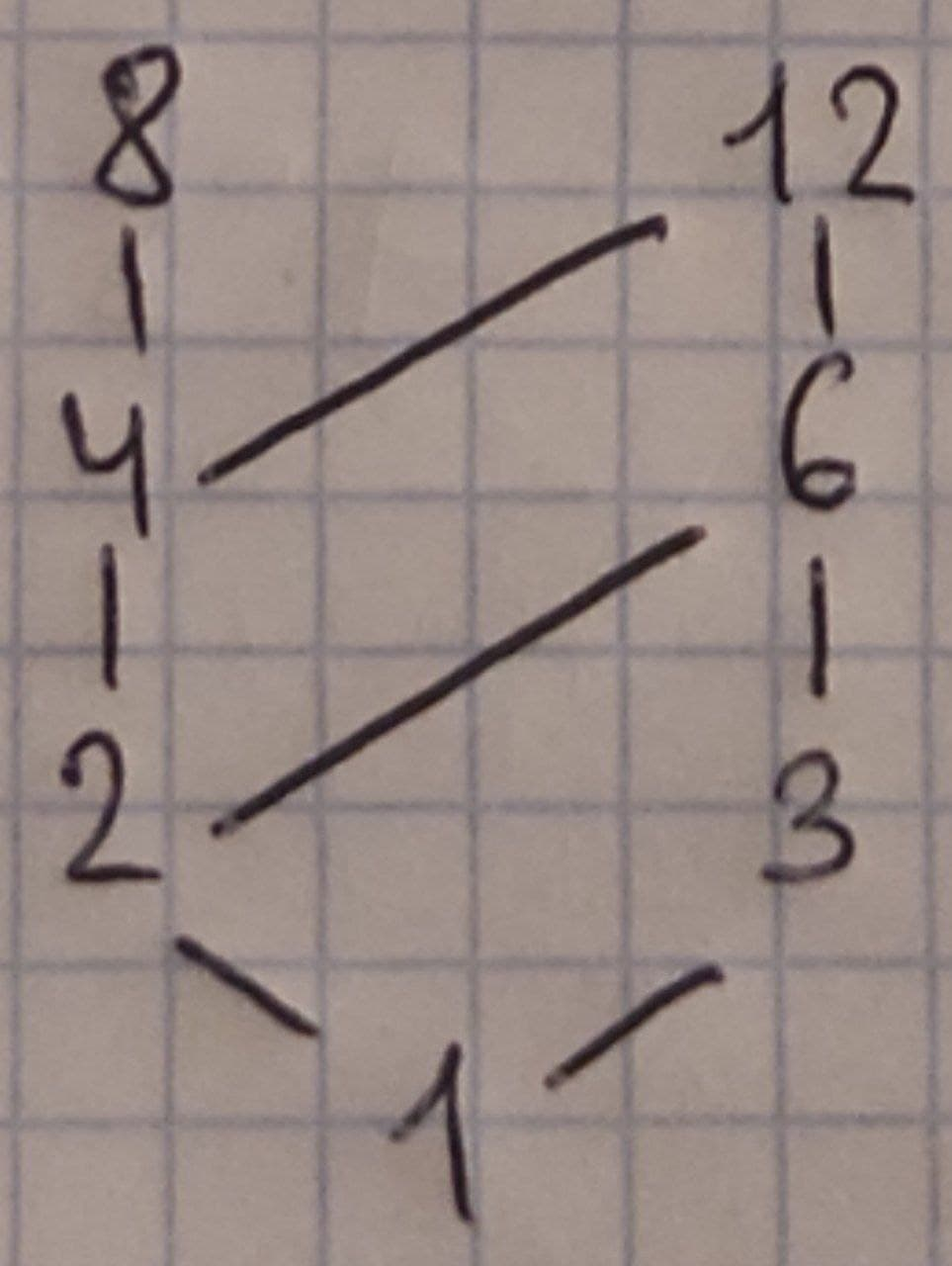2022-02-04

2.Construct the Hasse diagram that representing the Partial Ordering on {(a, b)| a divides b} on {1, 2, 3, 4, 6, 8, 12}.nick1337

Expert

Hasse diagram is a graphical representation of a partially ordered set. A={1, 2, 3, 4, 6, 8, 12}, the relation R="divisibility".

R={(1,1), (1,2), (1,3), (1,4), (1,6), (1,8), (1,12), (2,2), (2,4), (2,6), (2,8), (2,12), (3,3), (3,6), (3,12), (4,4), (4,8), (4,12), (6,6), (6,12), (8,8), (12,12)}.

Step 1. We construct a directed graph corresponding to a relation R.Step 2. We remove all loops from the diagram (reflexivity) and all transitive edges.Step 3. We make sure that the initial vertex is below the terminal vertex and remowe all arrows. See Hasse diagram:The minimal element is 1 (not preceeded by another element).

The maximal elements are 8 and 12 (not succeeded by another element).

The greatest element does not exist since there is no any one element that succeeds all other elements.

Do you have a similar question?